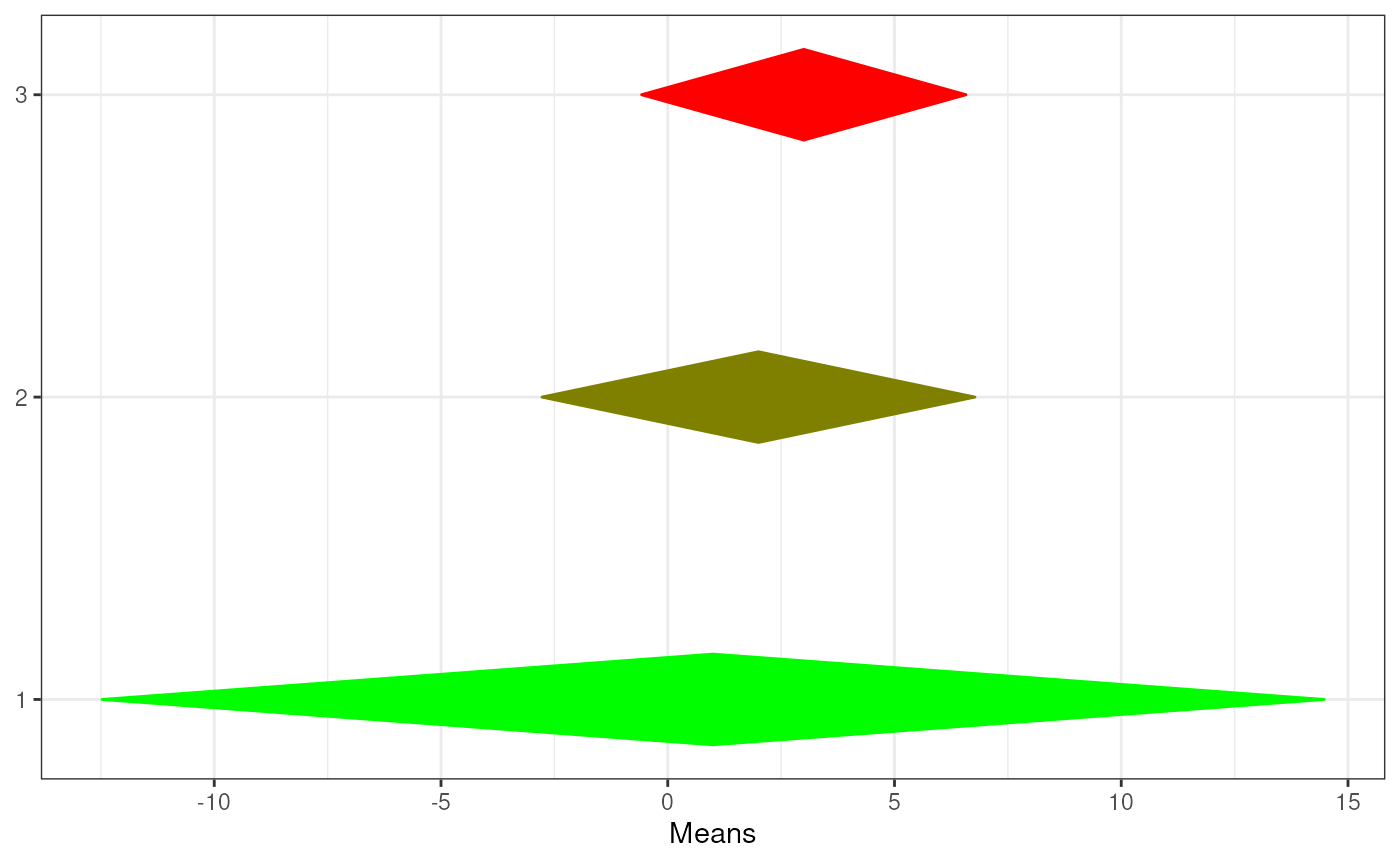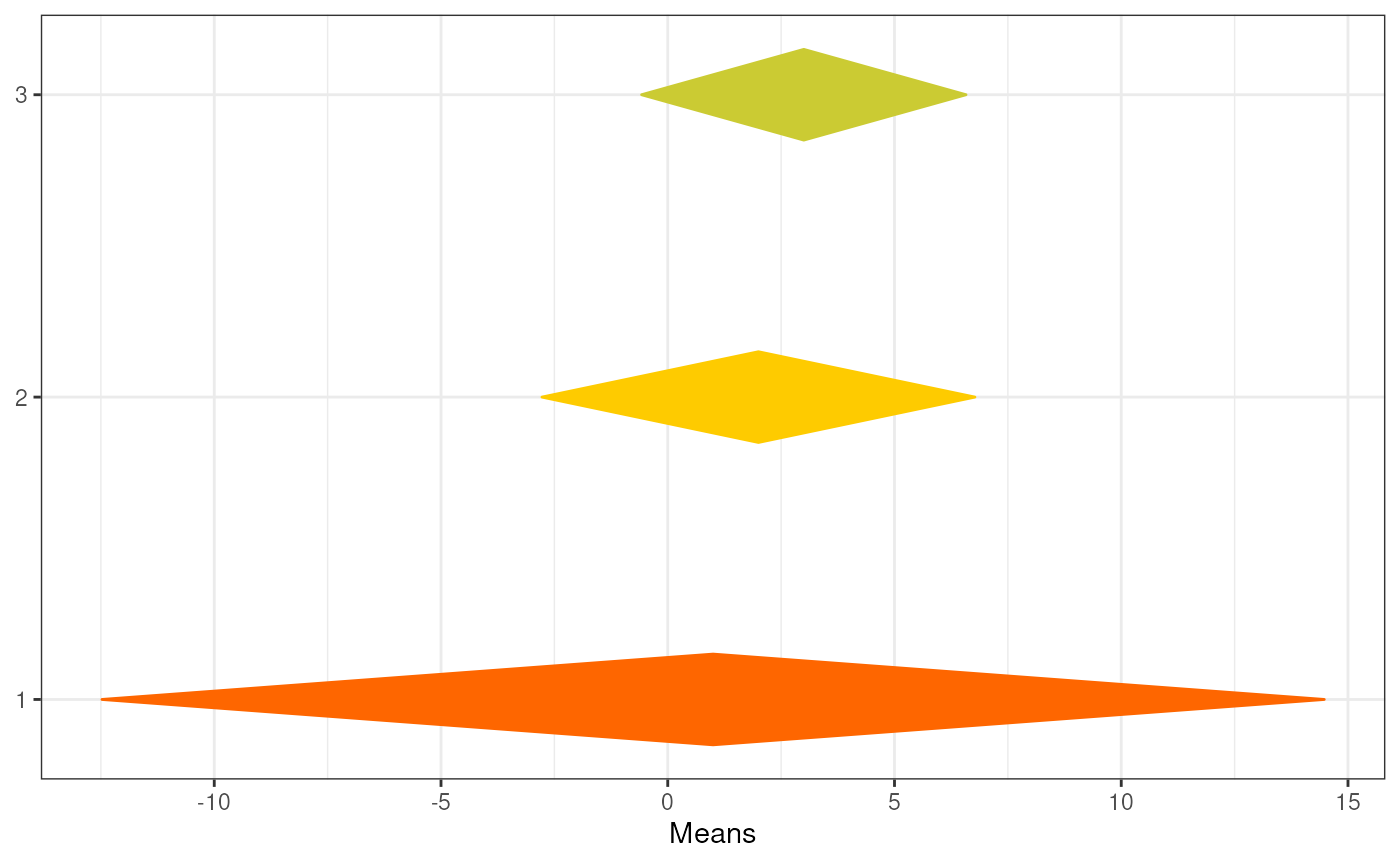This function generates a so-called diamond plot: a plot based on the forest plots that are commonplace in meta-analyses. The underlying idea is that point estimates are uninformative, and it would be better to focus on confidence intervals. The problem of the points with errorbars that are commonly employed is that the focus the audience's attention on the upper and lower bounds, even though those are the least relevant values. Using diamonds remedies this.

meanSDtoDiamondPlot(
dat = NULL,
means = 1,
sds = 2,
ns = 3,
labels = NULL,
colorCol = NULL,
conf.level = 0.95,
xlab = "Means",
outputFile = NULL,
outputWidth = 10,
outputHeight = 10,
ggsaveParams = ufs::opts\$get("ggsaveParams"),
...
)

## Arguments

dat

The dataset containing the means, standard deviations, sample sizes, and possible labels and manually specified colors.

means

Either the column in the dataframe containing the means, as numeric or as character index, or a vector of means.

sds

Either the column in the dataframe containing the standard deviations, as numeric or as character index, or a vector of standard deviations.

ns

Either the column in the dataframe containing the sample sizes, as numeric or as character index, or a vector of sample sizes.

labels

Optionally, either the column in the dataframe containing labels, as numeric or as character index, or a vector of labels.

colorCol

Optionally, either the column in the dataframe containing manually specified colours, as numeric or as character index, or a vector of manually specified colours.

conf.level

The confidence of the confidence intervals.

xlab

The label for the x axis.

outputFile

A file to which to save the plot.

outputWidth, outputHeight

Width and height of saved plot (specified in centimeters by default, see ggsaveParams).

ggsaveParams

Parameters to pass to ggsave when saving the plot.

...

Additional arguments are passed to diamondPlot() and eventually to ggDiamondLayer(). This can be used to, for example, specify two or more colors to use to generate a gradient (using generateColors and maybe fullColorRange).

## Value

A ggplot() plot with a ggDiamondLayer() is returned.

meansDiamondPlot(), diamondPlot(), factorLoadingDiamondCIplot(), ggDiamondLayer()

## Author

Gjalt-Jorn Peters

Maintainer: Gjalt-Jorn Peters gjalt-jorn@userfriendlyscience.com

## Examples


tmpDf <- data.frame(means = c(1, 2, 3),
sds = c(1.5, 3, 5),
ns = c(2, 4, 10),
labels = c('first', 'second', 'third'),
color = c('purple', 'grey', 'orange'));

### A simple diamond plot
meanSDtoDiamondPlot(tmpDf);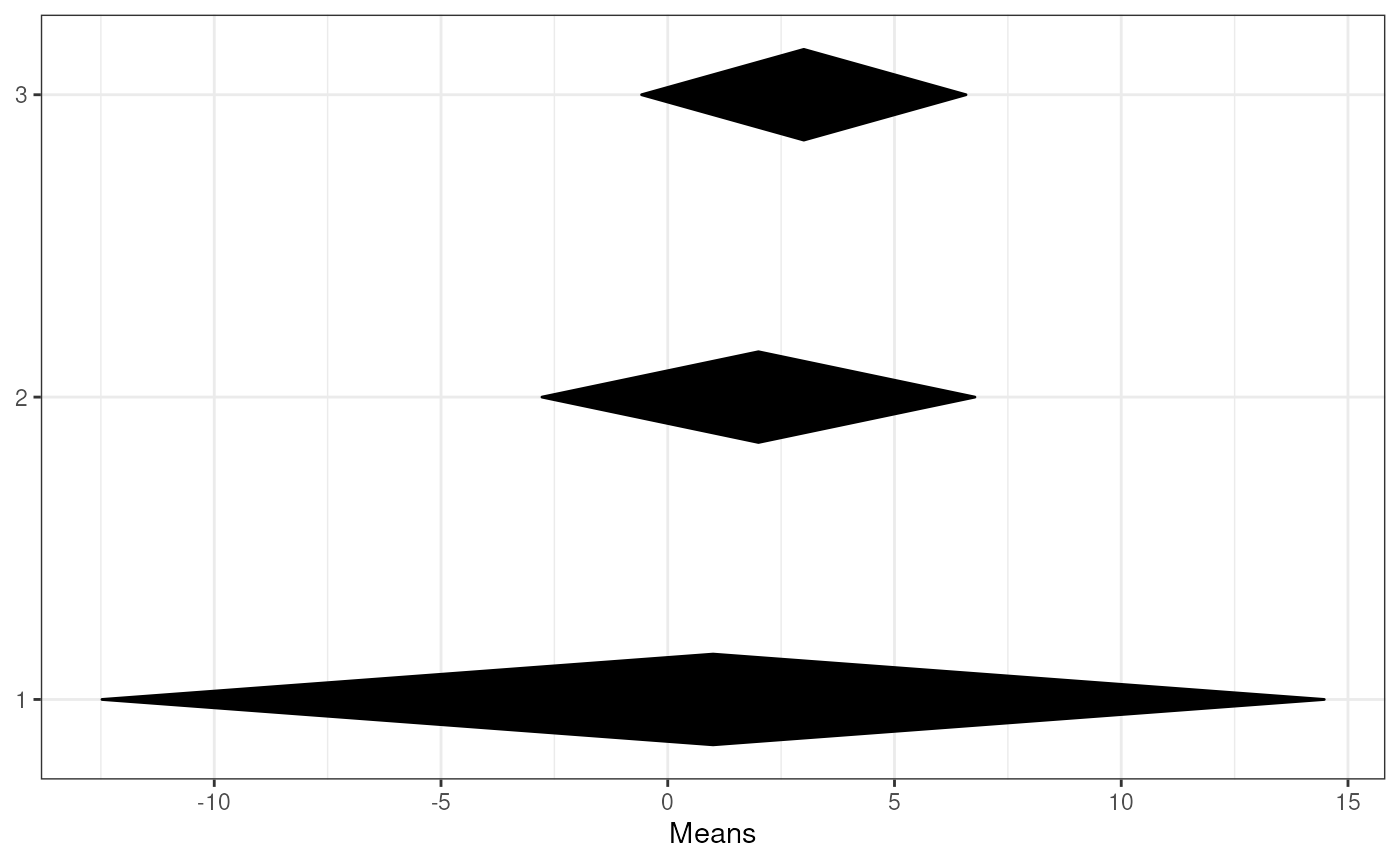### A simple diamond plot with labels
meanSDtoDiamondPlot(tmpDf, labels=4);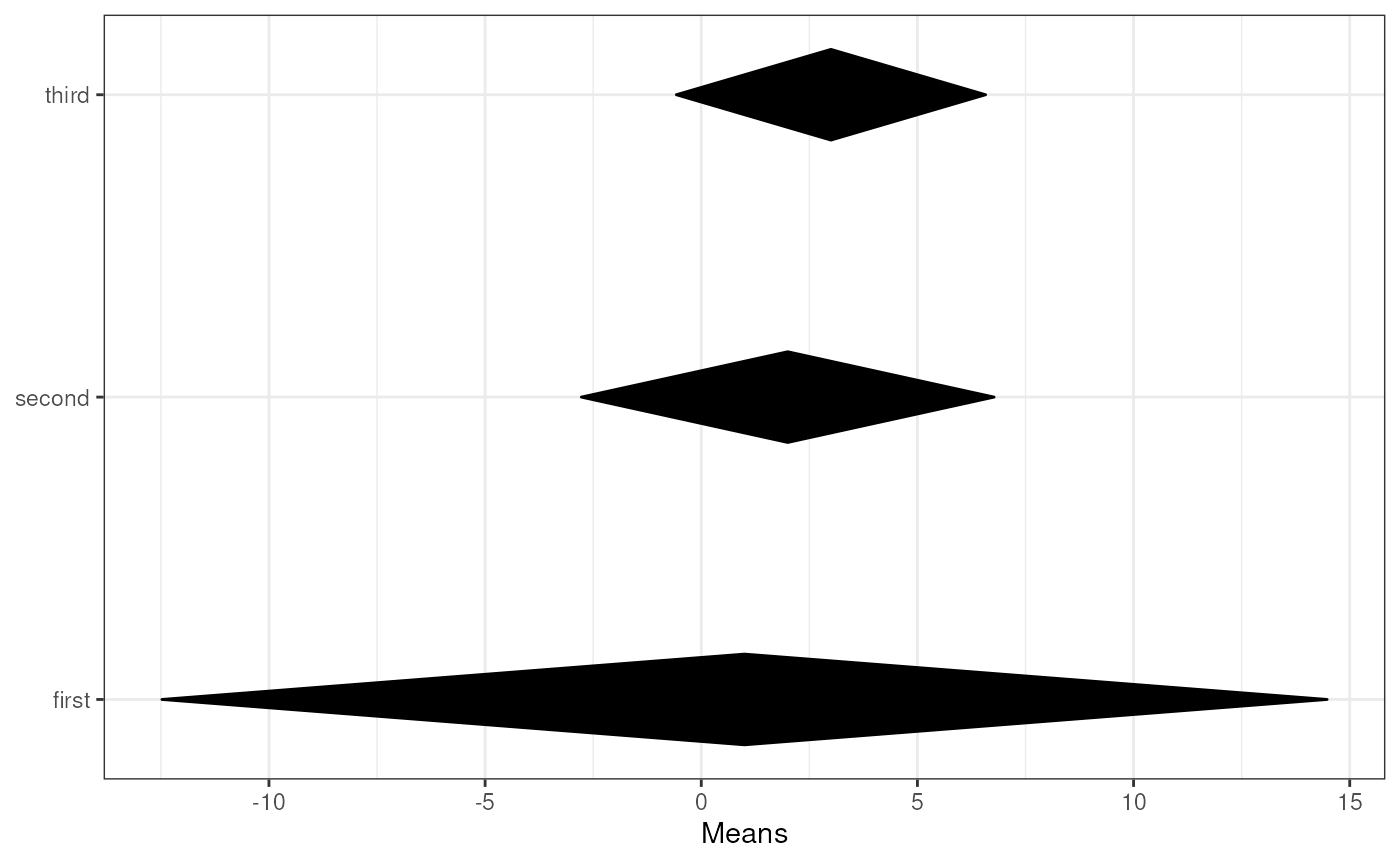### When specifying column names, specify column
### names for all columns
meanSDtoDiamondPlot(tmpDf, means='means',
sds='sds', ns='ns',
labels='labels');### A diamond plot using the specified colours
meanSDtoDiamondPlot(tmpDf, labels=4, colorCol=5);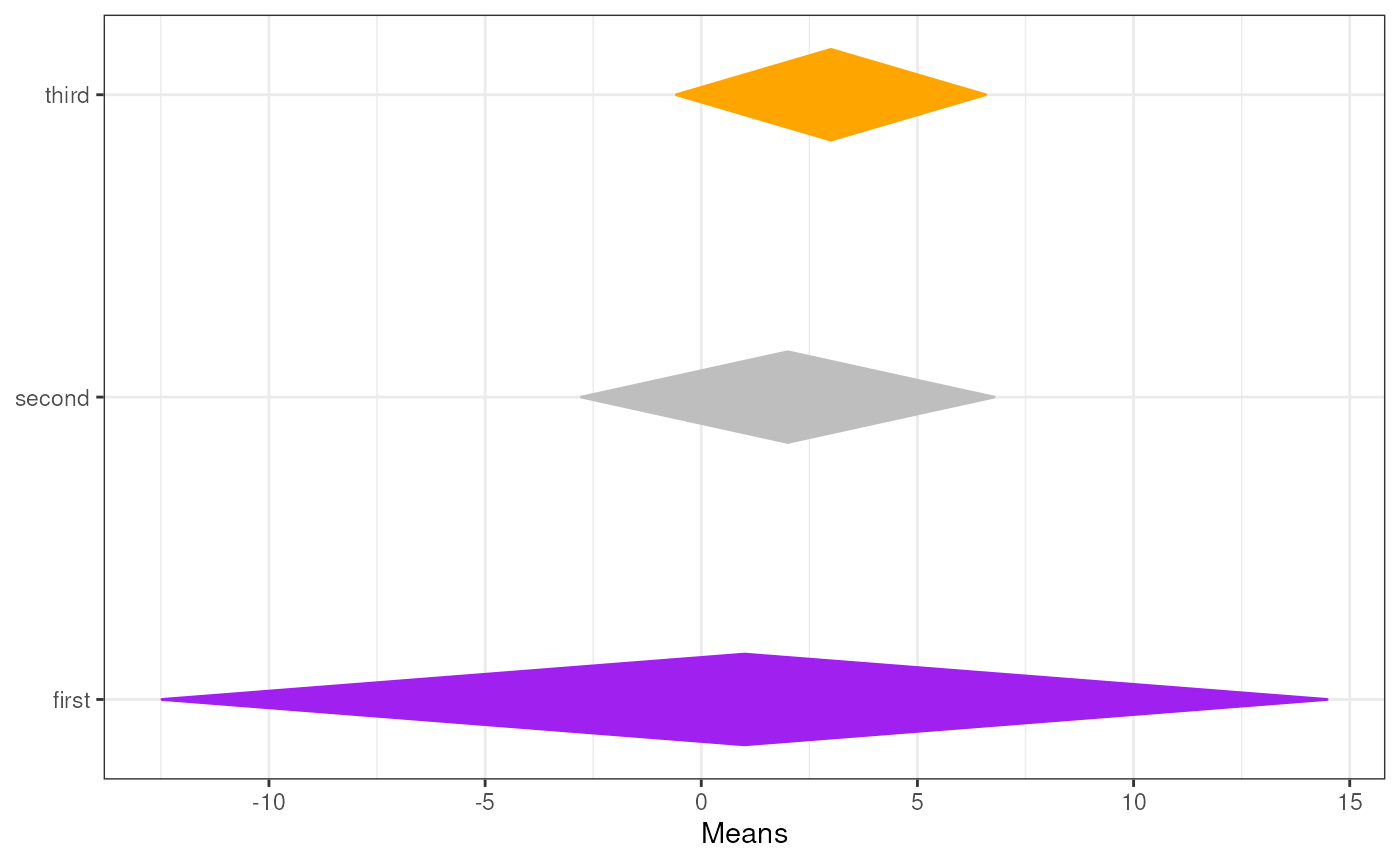### A diamond plot using automatically generated colours